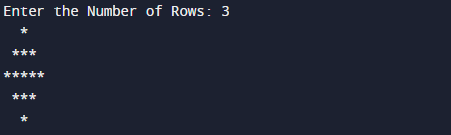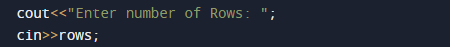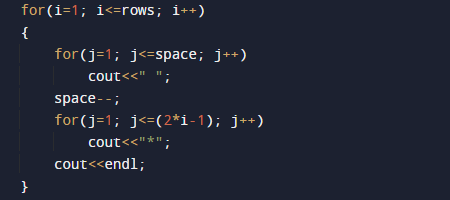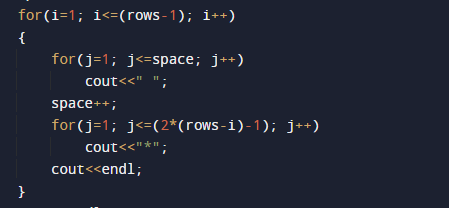# C++ Program to Print Diamond of Star

In this tutorial you will learn about the C++ Program to Print Diamond of Star and its application with practical example.

In this tutorial, we will learn to create a c++ program that will create Diamond of Stars (*) using c++ programming.

## Prerequisites

Before starting with this tutorial we assume that you are best aware of the following C++ programming topics.

• if-else Statements.
• Looping statement.
• Basic input/output.
• Operators.

## Diamond of Star.

Using C++ language to print diamond of stars, here we need to print two triangle one in upward direction and one in reverse, simply print first triangle and second triangle is reverse of first triangle.## C++ Program to Creating Diamond of Star.

In this program we will print Diamond of Star.Using nested  for loop. We would first declared and initialized the required variables. Then we will create Diamond of Star using below program.

## OutputIn the above program, we have first declared and initialized a set variables required in the program.• i & j= for  iteration.
• rows= for number of rows.
• space= for printing space.

Here  we will use two nested for loop First nested loop creates  upper triangular part  up-to 3 lineswhereas its lower triangular part  up-to 2 (one less than row size) lines.To print diamond pattern in our program, user will be prompted to enter the number of rows. Now using the row size we will print diamond pattern as shown in the program  below.In this tutorial we have learn about the C++ Program to Print Diamond of Star and its application with practical example. I hope you will like this tutorial.